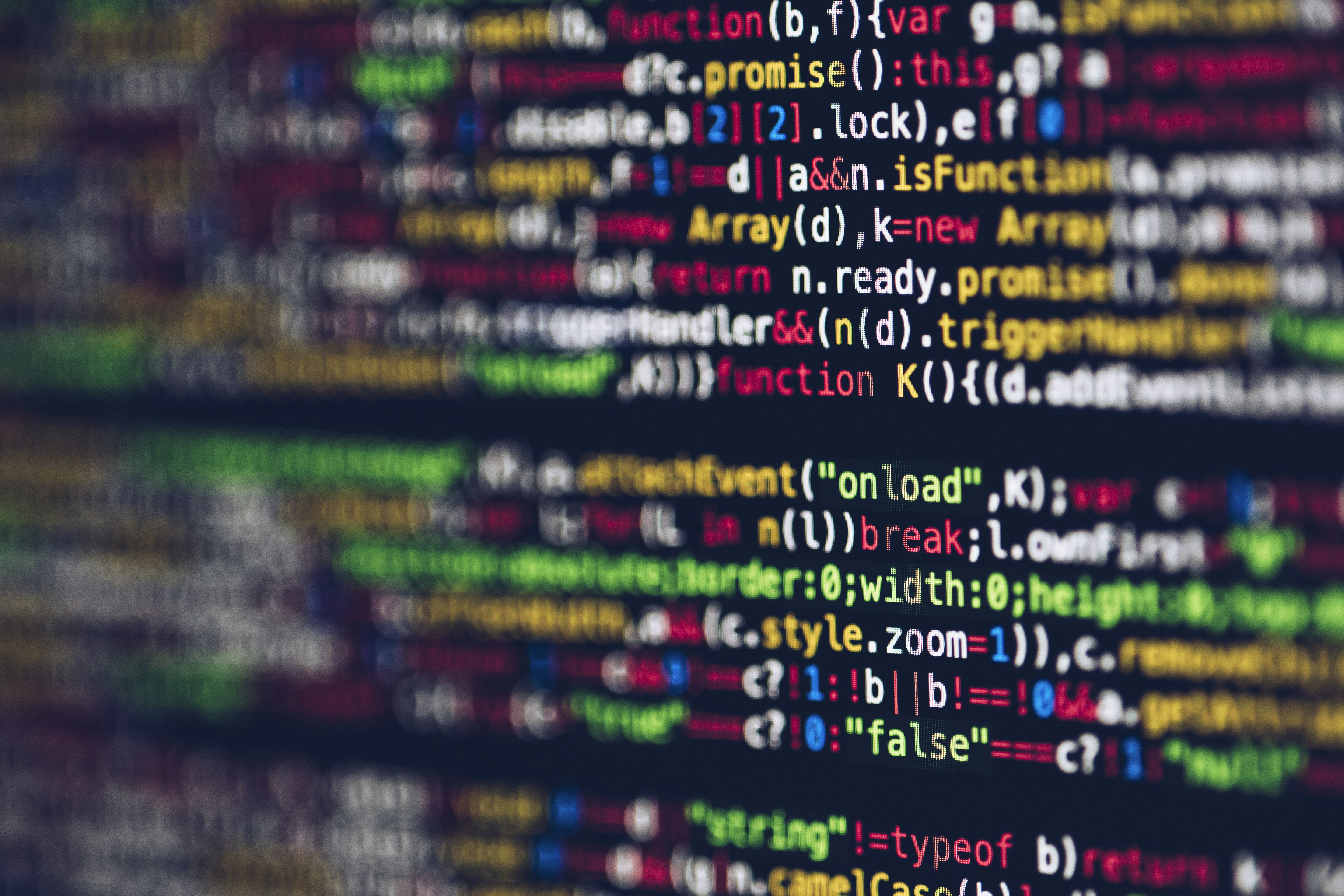Power of two

# Power of twoGiven an integer, write a function to determine if it is a power of two.

Example 1:

``````Input: 1
Output: true
Explanation: 2 pow 0 = 1
``````

Example 2:

``````Input: 16
Output: true
Explanation: 2 pow 4 = 16
``````

Example 3:

``````Input: 218
Output: false
``````

## Solution

If a number is power of 2, then its high bit is `1` and there is only one `1`. Therefore, `number & (number-1)` is `0`.

``````fun isPowerofTwo(n: Int): Boolean {
return (n>0).and((n and (n-1)) == 0)
}``````